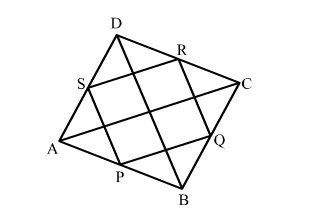# The diagonals of a quadrilateral ABCD are perpendicular to each other.

Question:

The diagonals of a quadrilateral ABCD are perpendicular to each other. Prove that the quadrilateral formed by joining the midpoints of its sides is a rectangle.

Solution:Given: In quadrilateral ABCDAC "> BDP, Q, R and S are the mid-points of AB, BC, CD and AD, respectively.

To prove: PQRS is a rectangle.

Proof:

In ΔABCP and Q are mid-points of AB and BC, respectively.

$\therefore P Q \| A C$ and $P Q=\frac{1}{2} A C$         (Mid-point theorem)          ...(1)

Similarly, in ΔACD,

So, R and S are mid-points of sides CD and AD, respectively.

$\therefore S R \| A C$ and $S R=\frac{1}{2} A C$     (Mid-point theorem)        ...(2)

From (1) and (2), we get

PQ || SR and PQ = SR

But this is a pair of opposite sides of the quadrilateral PQRS,

So, PQRS is parallelogram.

Now, in ΔBCDQ and R are mid-points of BC and CD, respectively.

$\therefore Q R \| B D$ and $Q R=\frac{1}{2} B D$       (Mid-point theorem)          ...(3)

From (2) and (3), we get

SR || AC and QR || BD

But, AC  BD      (Given)

∴ RS  QR

Hence, PQRS is a rectangle.